## Types of reaction

We will now look at the reaction level in more detail.  There are several ways to classify organic reactions, but it is helpful here for is to organize reactions in terms of the overall process that occurs.  There are three main types: Substitution, elimination and addition.

# Substitution

In this process, we simply replace one group (X) with another (Y).  We will initially be studying nucleophilic substitution (SN2 and SN1, chapters 8 & 9), where the “replacing” group Y is a nucleophile.  However, we can also have electrophilic substitution, where Y is an electrophile, and we will study this next semester (in aromatic substitution reactions).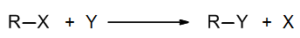Notice that here we are working at the reaction level, looking at the overall change in the reaction, not the details of what happens at the mechanism level.  For example, next semester we study the acyl substitution reaction, where the mechanism involves an addition followed by an elimination, leading to a substitution overall.

A typical example of nucleophilic substitution would be this SN2 reaction, where the Br (leaving group) is replaced by the OH (an incoming nucleophilic group):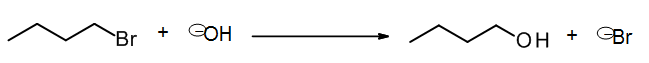which may be written in scheme format as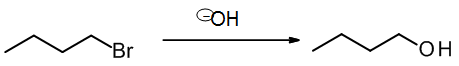We can summarize substitution in terms of the overall bond changes: We have broken one sigma bond (R-X or C-Br) and replaced it with a new sigma bond (R-Y or C-O).

# Elimination

Here, an organic molecule eliminates groups X and Y (which may leave either separately or together) to form a new pi bond.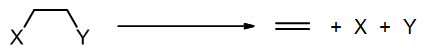The reaction may be driven by another molecule, such as an acid or base.  In the next chapter we will learn about two mechanisms that lead to formation of an alkene by an overall elimination reaction: E2 and E1, which are assisted by another molecule.  In other cases a different pi bond may be formed, such as C=O.  In this example E2 elimination, the formation of an alkene is driven by a base (hydroxide ion):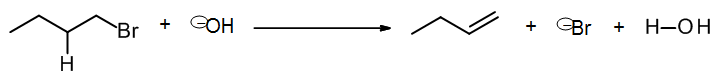which may be written as a scheme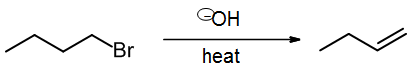Overall, we break two sigma bonds and form a new pi bond.  [A new sigma bond is also formed but is hidden, because it is the H-OH bond formed to produce the by-product, water (not shown).]

Addition is the opposite of elimination: X and Y add across a pi bond and form two new sigma bonds.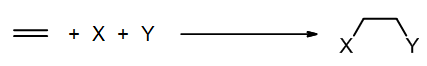In chapter 10 we will learn about a common type of addition called electrophilic addition, where an alkene reacts with an electrophile.  Next semester we will study nucleophilic additions to the pi bond of a carbonyl group (C=O).  In the following electrophilic addition example, X and Y are in the same molecule, HBr, which is the electrophilic agent.  This reaction can be written either as a balanced equation or a scheme: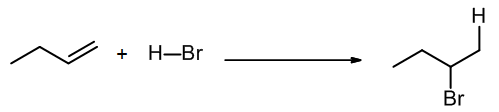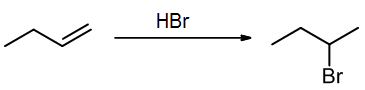Note that overall we lose a pi bond, and we form two new sigma bonds.  [Also, a sigma bond was broken but not shown explicitly, in H-Br bond of the reagent.]

### Common types of reaction

• Substitution: Replace sigma bond with another sigma bond
• Elimination: Break two sigma bonds and form a new pi bond
• Addition: Break a pi bond and form two new sigma bonds